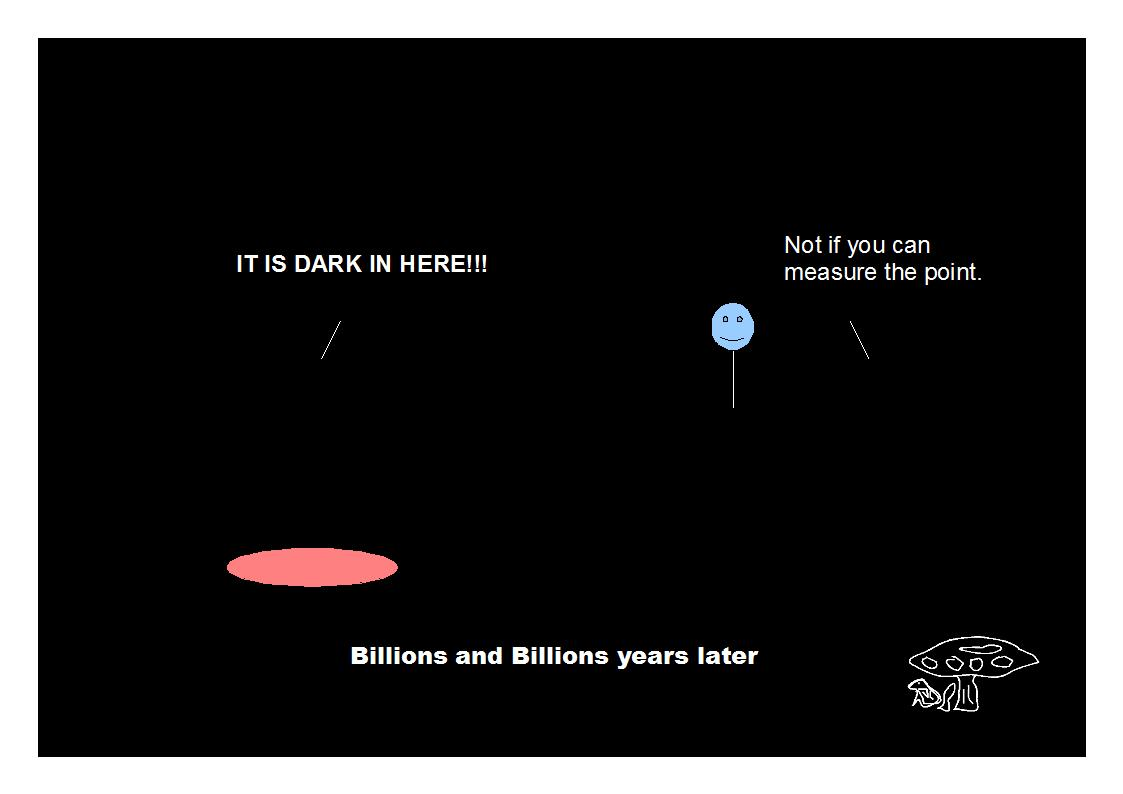# ijspace.org

 Force, Entropy, and Information Space 14th September 2014       We think of the force in terms of Newton's laws i.e. the force is the rate of change of momentum.  This definition is valid in an inertial-frame.  We know different types of forces namely Gravitational, Electromagnetic, and Nuclear.  The force allows the change in the state of a given system by overcoming the inherent inertia.  The force also maintains the structural integrity.  The forces between the entities require exchange of some information such as photons in the case of electromagnetic forces.  We also have gravitons, gluons, bosons and more to come, defining different types of interactions.  Currently our objective as it seems, is to develop the mechanism which allows us to combine these forces, a sort of reverse engineering approach.  Maybe we can try to take a different approach and try to think of a mechanism from which the forces that we know of, can be generated.  Let us try to develop a framework for the definition of force and then subsequently we will try to figure out if we can develop a description of the events we as humans experience, based on this framework.     The following assumes that a force represents an interaction, and an interaction represents a measurement. 1. A force can not exist without a measurement. 2. The nature of force is independent of the measurement metric, as a measurement metric is associated with the capability of the observer. 3. The strength of the force is proportional to the information content of the source being measured. 4. An observer will stay in orbit until the measurement is completed i.e. the force will exist until the measurement is completed. 5. If the observer's capability does not change during the course of measurement, the strength of interaction and associated force will diminish as the measurement progress. (All the measurements required to be completed, can not be completed.) 6. An observer can be in a measurement but may not know it as the observer may not be capable enough to measure the force associated with the measurement.       These definitions do not consider the force measurement values (magnitude and direction) which themselves are metric dependent along with the description of the entities by an observer such as physical bodies celestial or elementary, and their movements.  The existence of the measurement immediately puts the observer in the j-space.  We had discussed something similar in the introduction when we said,   "Every observer moves towards the state with maximum information, the observer can measure." If a state with infinite information is available but the observer can not measure it then the observer can not move towards it, however the observer will stay in orbit.         We can also think of the entropy of the measurements.  We note that the minimum number of measurements required to observe a PE1 event is one which means the entropy is in this case will be zero.  So a force can exist without entropy and possibly without time. In other words a force can be finite at the origin of the event.  We can also think of it as the force required to make a measurement by an observer with ∞j  capability i.e. an observer who can observe an event in quantum domain with a single measurement.  In q =3 state it will be an observer with capability to make measurements in Planck's domain.  The quantities in the right side of the force equation (F = dp/dt or f(m,r,t)) such as mass, time, momentum etc. come into picture only with entropy.  It is also very important to understand that the moment we introduce entropy which is the characteristics of the j-space, the VT-Symmetry  is implicit.  We had earlier described the Infinite Fulcrum, which is based on the definitions provided above.  The Infinite Fulcrum applies to the celestial and the elementary structures both.Information on www.ijspace.org is licensed under a Creative Commons Attribution 4.0 International License.